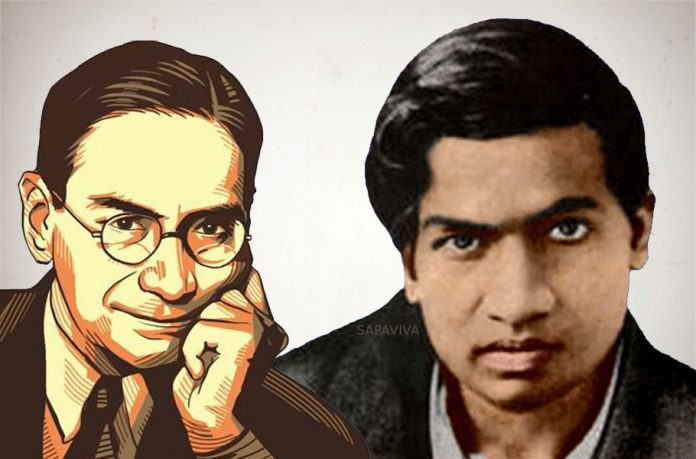# When two geniuses meet: Ramanujan, Mahalanobis, and a Sunday puzzle

There are several anecdotal stories about the two men from their time at Cambridge. Here is one that partially explains the genius of RamanujanSrinivasa Ramanujan, the self-made mathematical genius of Kumbakonam, went to Cambridge at the invitation of Professor G.H. Hardy for his research. Ramanujan’s contemporary there was none other than P.C. Mahalanobis, a member of the first Planning Commission of India and founder of the Indian Statistical Institute.

When they met both men were in their 20s and considered to be geniuses. There are many anecdotal stories about them in the book The Man Who Knew Infinity, Robert Kanigel’s biography of Ramanujan. Here is one that partially explains the genius of Ramanujan.

The Strand was a monthly London-based magazine that published short stories and popular nonfiction in the late 19th and early 20th Century. It is perhaps best known nowadays for publishing Arthur Conan Doyle’s Sherlock Holmes stories. It was also well known for its puzzle column.

AdvertisementMahalanobis came to Ramanujan one Sunday morning clutching a copy of the Strand. Ramanujan was in his room making tea. (Why tea and not Kumbakonam ‘degree coffee’?) Their conversation went like this:

M: There is an interesting puzzle here in these pages. I am happy to have solved it with some difficulty. Would like your comments.

R: Go ahead and tell me the puzzle.

M: It goes like this: There is a street with houses numbered sequentially from 1. The number of houses is more than 50. My friend is living in a house somewhere in the middle such that if you add the numbers before and after that house the sums are equal. Tell me how many houses are there in the street, and which house my friend lives in.

M: For example, if there are 8 houses and my friend is on house number 6, it will agree, because:

1 + 2 + 3 + 4 + 5 = 15 = 7 + 8

After much trial and error, I could get the answer:

Number of Houses          Friends House

289                            204

M: What do you think?

R, after thinking for a few minutes and mixing his tea in between: Interesting. But that is not the only answer. There are infinite number of answers. Take down some of them.M: I am shocked. How did you find them?

R: Actually, it is not that difficult. I realised there must be a continued fraction, which when truncated will have a numerator and denominator, which will give us the number of houses and the friend’s house. The question is which continued fraction? There is a simple answer like ‘8’ and ‘6’. We can write another simple answer, like ’49’ and ’35’. Finally you gave me ‘289’ and ‘204’. That gave me the clue. And then the answers came. The continued fraction needed is:Ramanujan was right. He was ingenious enough to guess the answer immediately. But how did he do it? This will require some school-level maths.

Let us assume ‘N’ is the number of houses and ‘M’ is the friend’s house number. Using the formula to calculate the sum of integers up to N (taught in schools)…Today such equations are called ‘Fermat-Pell Equation’. But our own Brahmagupta (598-668 CE) discovered them 1,000 years ago!

So if we know x and y, then we can get N and M, which solves the problem posed by Mahalanobis. That is where the ingenuity of Ramanujan comes. If x, y are large then the number 1 is too small and we can approximate:But we know 2 is irrational number and y, x are integers. Hence we cannot write 2 as a fraction. But we can write it as a continued fraction and to account for dropping 1 we can truncate and get the answers.

The truncated values are:…as a fraction. It is easy to write down the above fractions since there is a pattern. Each one of the above give approximations for 2.

Now we can get easily N,M as…

(N, M ) = (1, 1), (8, 6), (49, 35), (289, 204), (1681, 1189), (9800, 6930)…

The first solution is trivial. The second and third can be found by trial and error. But the number of houses is not more than 50. The fourth is what Mahalanobis found. And the others and infinite number of them were given by Ramanujan.

We can now understand the great ingenuity of Ramanujan. Now we will complete with interesting information about Brahmagupta-Fermat-Pell equation.

Brahmagupta gave an equation like (with x, y as integers)

Nx2 + 1 =  y2

And he also gave a method for solving them. When N = 2 we get the previous equation whose solutions were obtained by Ramanujan. But Brahmagupta asked to solve when N = 61. That is, get an integer solution for

61x2 + 1 =  y2

Even Ramanujan could not solve easily by his method! This equation was discovered again by Fermat-Pell 1,000 years later. Fermat challenged many mathematicians to solve the above and many top-level mathematicians failed. But it was solved by Bhaskara II around 1150 CE! The difficulty in this problem was because the first integer solution was

(x, y) = (226153980, 1766319049)

Squaring such numbers require more than calculators or personal computers. It is not possible to arrive at through simple guess work or trial and error. One needs solid algorithms. One such algorithm, known as the Chakravala method, was used by Bhaskara.

The writer was a professor, Institute of Mathematical Sciences Chennai.